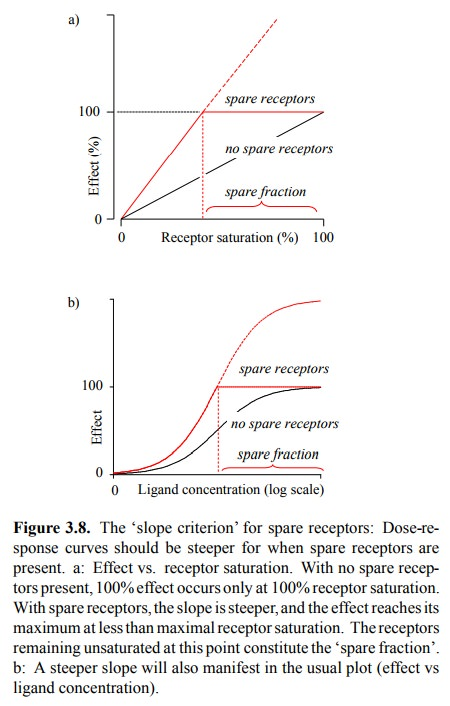Home | | Biochemical Pharmacology | Spare receptors

# Spare receptors

If, as in the above example, some of the receptors can be in-activated without a decrease in the maximal effect, the dis-pensble receptor fraction is commonly referred to as `spare receptors'.

Spare receptors

If, as in the above example, some of the receptors can be in-activated without a decrease in the maximal effect, the dis-pensble receptor fraction is commonly referred to as `spare receptors'. Despite its widespread use in the literature, the term is not very precisely defined, and some argument ex-ists about its proper use. Some authors consider a parallel shift of the dose-effect curve in response to an irreversible inhibitor (as for curves 1 and 2 in Figure 3.9) sufficient evi-dence of spare receptors. Using this interpretation, it would seem that any system with an initial gap between dose-ef-fect and dose-receptor occupancy curves would qualify. Others insist that not only should there be a gap between dose-effect and dose-receptor occupancy curves, but that also the dose-effect curve should be steeper than the dose-receptor occupancy curve.The second position can be summarized as follows: With or without spare receptors, each occupied receptor molecule should make the same contribution toward the effect until the maximum effect is reached. Therefore, with spare re-ceptors present, 100% of the effect should be reached with less than 100% of the receptors occupied, which means that the response curve for the function must be steeper than that for the receptor occupancy (Figure 3.8). Note that this argument assumes a linear relationship between receptor binding and functional effect. I therefore think that the slope criterion is not generally applicable.Study Material, Lecturing Notes, Assignment, Reference, Wiki description explanation, brief detail
Biochemical Pharmacology : Pharmacodynamics : Spare receptors |